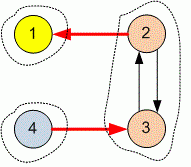Competitions

# Condensation of the graph

Find the number of edges in the condensation of a given directed graph.

The condensation of a directed graph G is a directed graph G', whose vertices are strongly connected components of G, and the edge in G' is present only if there exists at least one edge between the vertices of corresponding connected components.

The graph condensation does not contain multiple edges.

#### Input

The first line contains number of vertices n and number of edges m (n104, m105) in the graph. Each of the next m lines describe the edge of the graph. The i-th edge is given with the starting bi and the ending ei (1bi, ein) vertex of the graph. The input graph can contain the multiple edges and loops.

#### Output

Print the number of edges in graph condensation.Time limit 2 seconds
Memory limit 128 MiB
Input example #1
4 4
2 1
3 2
2 3
4 3

Output example #1
2

Author Vitaly Goldstein
Source Winter School, Kharkov, 2011, Day 9